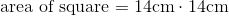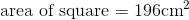# ISEE Lower Level Math : How to find the area of a square

## Example Questions

### Example Question #31 : How To Find The Area Of A Square

What is the area of a square with a side length of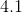inches?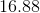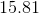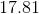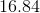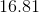Explanation:

To find the area of a square, simply multiply the side length by itself. Asis the side length, that is the number you will use. The best answer is: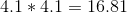### Example Question #32 : How To Find The Area Of A Square

What is the area of a square with a side length of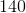inches?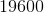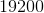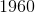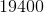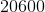Explanation:

To find the area of a square, simply multiply the side length by itself. Asis the side length, that is the number you will use. The best answer is: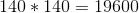### Example Question #33 : How To Find The Area Of A Square

Find the area of a square with a side having a length of 9 inches.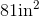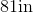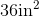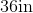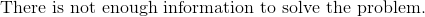Explanation:

To find the area of a square, we use the following formula: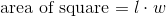where l is the length and w is the width of the square.

We know the square has a side of length 9 inches.  We also know a square has 4 equal sides.  So all sides are the same.  Knowing this, we can substitute into the equation.  We get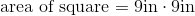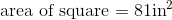### Example Question #34 : How To Find The Area Of A Square

Find the area of a square with a length of 6in.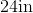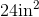Explanation:

To find the area of a square, we will use the following formula:where l is the length and w is the width of the square.

Now, we know the length of the square is 6in.  Because it is a square, we know that all sides are equal and all sides have the same length.  Therefore, the width is also 6in.  Knowing this, we can substitute into the formula.  We get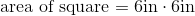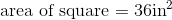### Example Question #35 : How To Find The Area Of A Square

Find the area of a square with a base of length 3 feet.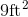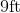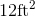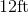Explanation:

To find the area of a square, we will use the following formula:where l is the length and w is the width.

We know the square has a base of length 3 feet.  Because it is a square, we know that all sides are equal.  This means that all sides have the same length.  Therefore, the width is also 3 feet.  Knowing this, we can substitute into the formula.  We get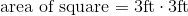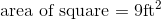### Example Question #1191 : Isee Lower Level (Grades 5 6) Mathematics Achievement

Find the area of a square with a base of length 3 feet.Explanation:

To find the area of a square, we will use the following formula:where l is the length and w is the width of the square.

Now, we know the base, or length, of the square is 3 feet.  Because it is a square, we know that all sides are equal.  Therefore, the width is also 3 feet.  Knowing this, we can substitute into the formula.  We get### Example Question #1192 : Isee Lower Level (Grades 5 6) Mathematics Achievement

Find the area of square that has a width of 11cm.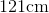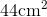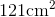Explanation:

To find the area of a square, we will use the following formula:where l is the length and w is the width of the square.

Now, we know the width of the square is 11cm.  Because it is a square, we know that all sides are equal, which means all sides are 11cm.  Therefore, the length is also 11cm.  Knowing this, we can substitute into the formula.  We get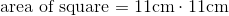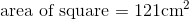### Example Question #1193 : Isee Lower Level (Grades 5 6) Mathematics Achievement

Find the area of a square with a width of 8in.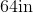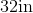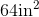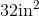Explanation:

To find the area of a square, we will use the following formula:where l is the length and w is the width of the rectangle.

Now, we know the width of the square is 8in.  Because it is a square, all sides are equal.  This means all sides are 8in.  Therefore, the length is 8in.  Knowing this, we can substitute into the formula.  We get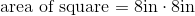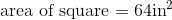### Example Question #31 : How To Find The Area Of A Square

Use the following square to answer the question: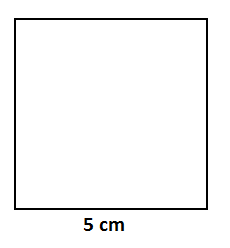Find the area.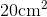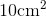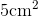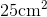Explanation:

To find the area of a square, we will use the following formula:where l is the length and w is the width of the square.

Now, let's look at the square.We can see that the length is 5cm.  Because it is a square, we know that all sides are equal.  Therefore, the width is also 5cm.

Knowing this, we can substitute into the formula.  We get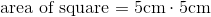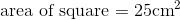### Example Question #1195 : Isee Lower Level (Grades 5 6) Mathematics Achievement

Use the following square to answer the question: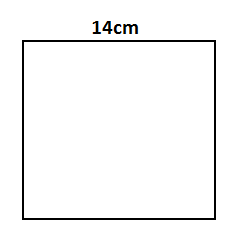Find the area.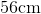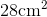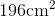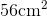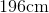Explanation:

To find the area of a square, we will use the following formula:where l is the length and w is the width of the square.

Now, given the squarewe can see the length is 14cm.  Because it is a square, all sides are equal.  Therefore, the width is also 14cm.

Knowing this, we can substitute into the formula.  We get